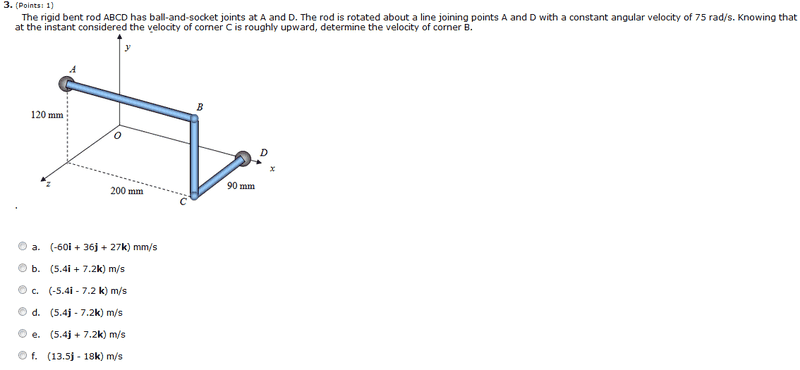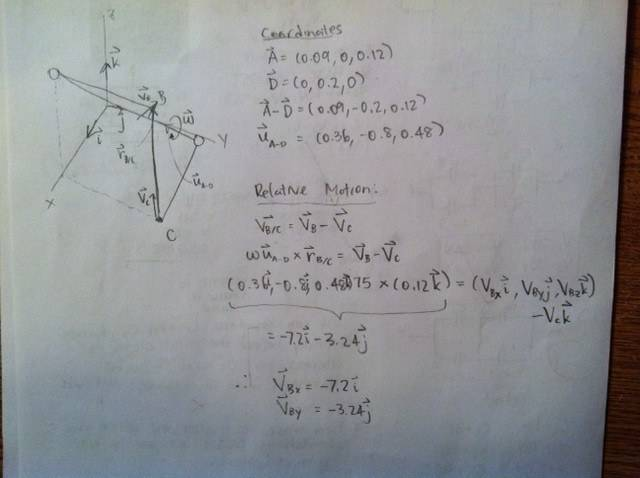# Relative motion with angular velocity

theBEAST

## Homework Statement## The Attempt at a Solution

Do note I switched up the i, j and k vectors.I am getting half the answer correct. -7.2 is correct according to the answer key but my other solution -3.24 is clearly wrong. Does anyone know what I did wrong? I tried to be neat as possible, if anyone is not able to follow please ask me about what I did.

Thanks!

klawlor419
Check your initial vectors defining the points A and D in the problem. Your vectors don't match with the picture defining the problem. So the components your getting are likely wrong.

theBEAST
Check your initial vectors defining the points A and D in the problem. Your vectors don't match with the picture defining the problem. So the components your getting are likely wrong.

Where did I make the mistake :S I don't see why A and D are wrong.

klawlor419
Look at the x-y-z axes in the picture you drew by hand and the axes in original picture of the problem! And remember that rotation is about the diagonal, connecting the ball bearings and that v = ω cross r

This should be enough to solve the problem

theBEAST
Look at the x-y-z axes in the picture you drew by hand and the axes in original picture of the problem! And remember that rotation is about the diagonal, connecting the ball bearings and that v = ω cross r

This should be enough to solve the problem

Okay I just redid it with
A = (0, 0.12, 0.09)
D = (0.2, 0 , 0)
A-D = (-0.2, 0.12, 0.09)
unit_vector_A-D = (-0.8, 0.48, 0.36)

Then I repeated the steps I took in the Relative Motion section of my answer and I got the same thing except this time I had -3.24i -7.2k. I think maybe my r_B/C vector is wrong? I am not sure.

So I switched the axis so it would match with the question but it seems like it doesn't matter.

Last edited:
klawlor419
Try this, so you now have the vector w determined. Ok good, now calculate the distance if point B off the axis of rotation. Now take the cross product. Don't worry about relative velocity, I don't think this problem needs to consider that.

theBEAST
Try this, so you now have the vector w determined. Ok good, now calculate the distance if point B off the axis of rotation. Now take the cross product. Don't worry about relative velocity, I don't think this problem needs to consider that.

Thanks! I was able to solve this problem by finding that vector from B to the axis of rotation and then taking this vector (r) and crossing it with the angular velocity vector w.

Does anyone know where I can learn about v = w x r? I am still a bit confused about why you use the cross product in this equation. These concepts are new to me and are concepts I must learn on my own. I tried to find a good explanation in the textbook but with no success.

klawlor419
Wikipedia has a decent article on cross products. Taylors Classical mechanics is good too